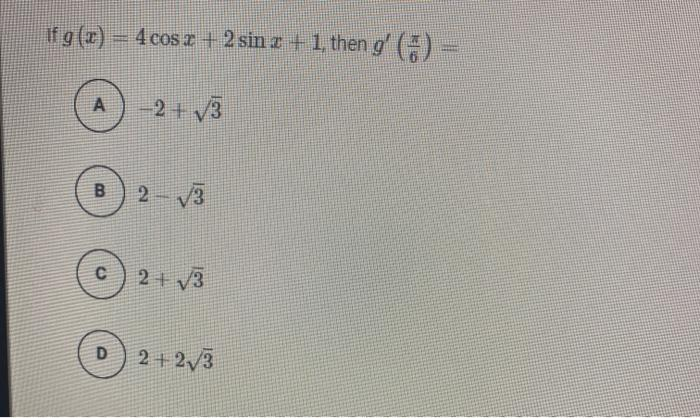### Create an Account

Already have account?

### Forgot Your Password ?

Home / Questions / if g(x) = 4cos 2 + 2 sin = + 1. then gʻ ( A - 2 + 13 B. 2 - V3 с 2 +V3 D 2 + 273

# if g(x) = 4cos 2 + 2 sin = + 1. then gʻ ( A - 2 + 13 B. 2 - V3 с 2 +V3 D 2 + 273

if g(x) = 4cos 2 + 2 sin = + 1. then gʻ ( A - 2 + 13 B. 2 - V3 с 2 +V3 D 2 + 273Apr 15 2021 View more View Less

#### Answer (Solved)Subscribe To Get Solution